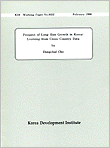## 본문

### KDI연구

KDI연구원들이 각 분야의 전문보고서를 제공합니다.

#### 거시

Working Paper

Prospect of Long-Run Growth in Korea : Learning from Cross-Country Data• 저자 조동철(曺東徹)
• 발행일 1996/02/01
• 시리즈 번호 9602
원문보기
요약 Predicting long-run growth pats using the Solow-like
growth formulas involves and inevitable problem : how to
predict dynamic paths of the respective explanatory variables.
This paper approacher this issue in the following four steps:
1) Estimate the relevant coefficients (elasticities) of
explanatory variables from cross-country regressions;
2) Using cross-country data, estimate the general pattern of
each explanatory variables as a (nonparametric) function
of income per capita;
3) Estimate Korea's country-specific effects in the
explanatory variables;
4) Using the above estimates, simulate the Korea's and
explanatory variables' paths simultaneously.
##### 저자조동철

저자의 다른 보고서
전체이동
##### 같은 주제 자료 이 내용과 같은 주제를 다루고 있는 자료입니다.

※문의사항 미디어운영팀 윤정애 전문연구원 044-550-4450 yoon0511@kdi.re.kr

가입하신 이동통신사의 요금제에 따라
데이터 요금이 과다하게 부가될 수 있습니다.

파일을 다운로드하시겠습니까?
KDI 연구 카테고리
상세검색